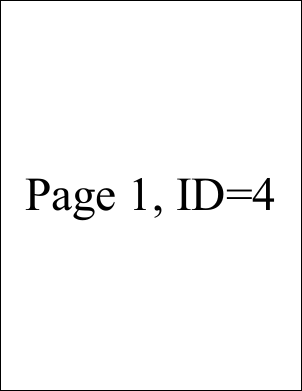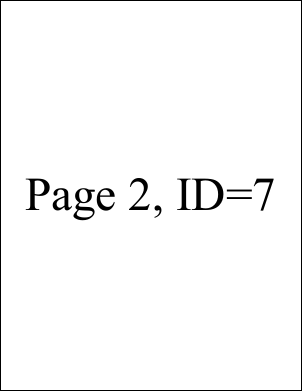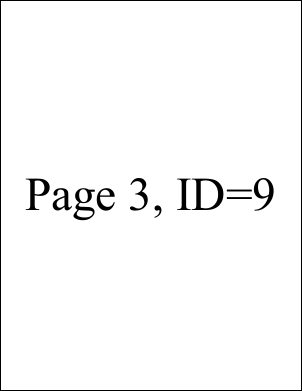AddPage FunctionAdds a page to the current document.Syntax

 ```int AddPage(); int AddPage(int page); ``` ```Function AddPage() As Integer Function AddPage(page As Integer) As Integer ```Params

 Name Description page The page insertion location. By default, pages are added at the end of the document. return The Object ID of the newly added Page Object.Notes

 Adds a page to the current document. The AddPage function returns the Object ID of the newly added Page Object. Typically, you will want to assign this return value to the document Page property using code of the form.   ```theDoc.Page = theDoc.AddPage(); ``` ```theDoc.Page = theDoc.AddPage() ``` Pages are added at the end of the document. However, you can use the page parameter to insert pages at other locations. The following code inserts a page at the start of a document.   ```theDoc.Page = theDoc.AddPage(1); ``` ```theDoc.Page = theDoc.AddPage(1) ``` Any existing page and all subsequent pages will be shifted towards the end of the document to make room for the insertion.Example

 The following code adds three pages to a document. Each page is marked with the page number and page Object ID.   ```using (Doc doc = new Doc()) {   doc.FontSize = 96; // big text   doc.TextStyle.HPos = 0.5; // centered   doc.TextStyle.VPos = 0.5; // ...   for (int i = 1; i <= 3; i++) {     doc.Page = doc.AddPage();     string txt;     txt = \$"Page {i}, ID=";     txt = txt + doc.Page.ToString();     doc.AddText(txt);   }   doc.Save(Server.MapPath("docaddpage.pdf")); } ``` ```Using doc As New Doc()   doc.FontSize = 96   ' big text   doc.TextStyle.HPos = 0.5   ' centered   doc.TextStyle.VPos = 0.5   ' ...   Dim i As Integer = 1   While i <= 3     doc.Page = doc.AddPage()     Dim txt As String     txt = \$"Page {i}, ID="     txt = txt + doc.Page.ToString()     doc.AddText(txt)     System.Math.Max(System.Threading.Interlocked.Increment(i),i - 1)   End While   doc.Save(Server.MapPath("docaddpage.pdf")) End Using ```docaddpage.pdf